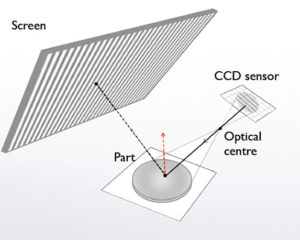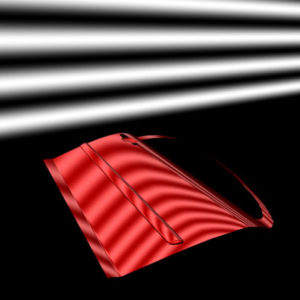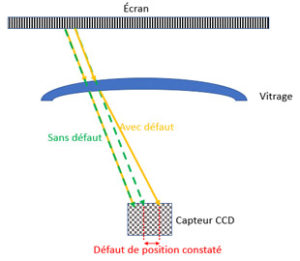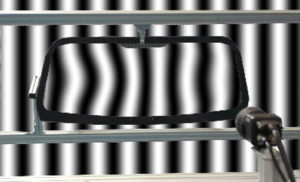# Phase shiftingdeflectometry technology

## V-Optics systemsare based on phase shifting deflectometry technology

### Deflectometry used in reflection (reflective surfaces):

The principle of phase shafting deflectometry (PSD) is to analyze the deformation of a perfect pattern reflected by the surface to be controlled.

On a perfect surface, we know in advance what would be the reflection of this perfect pattern and so we know where the light rays should go. If they are not where they are supposed to be, it means that the reflection of the rays has been modified by a local surface defect (variation of the surface slopes).

This principle is demonstrated by the law of Snell-Descartes which says that the reflection of the light rays is such that the angle of incidence and the angle of reflection are equal.• The algorithm reconstructs the path of the light rays coming from the screen pattern and reflected back to the object by the camera.
• The direction of the normal at any point of the surface is deduced and thus leads to the slopes.
• The curvatures of the surface can be obtained by deriving from the slopes.
• Slope integration leads to surface altitudes.• A dynamic sinusoidal pattern is broadcast on a screen
• The reflected pattern is distorted by the analyzed surface
• Information digitized by camera (s)
• The algorithm processes the deformation of the pattern

The advantage of PSD is that it is very sensitive to local altitude deviations.
This makes it possible to highlight the defects and characteristics of the surface.

### Deflectometry used in transmission (transparent products)

To qualify a transparent material, the analysis focuses on measuring the refraction of the object under test. Imperfections in the material or its surface cause changes in refraction and ability to transmit light.• The position of each emission point on the screen being known, the algorithm is able to detect the deviation of the light rays.
• The magnification between the perfect pattern and the captured image is calculated at each measurement point in the x and Y directions
• Optical power is deduced from magnification
• Optical distortions are calculated from its optical power• A dynamic sinusoidal pattern (in x and in y) is broadcast on a screen
• The pattern seen through the material is distorted by the various defects or characteristics of the part
• Information digitized by camera (s)
• The algorithm deals with the deformation of the pattern